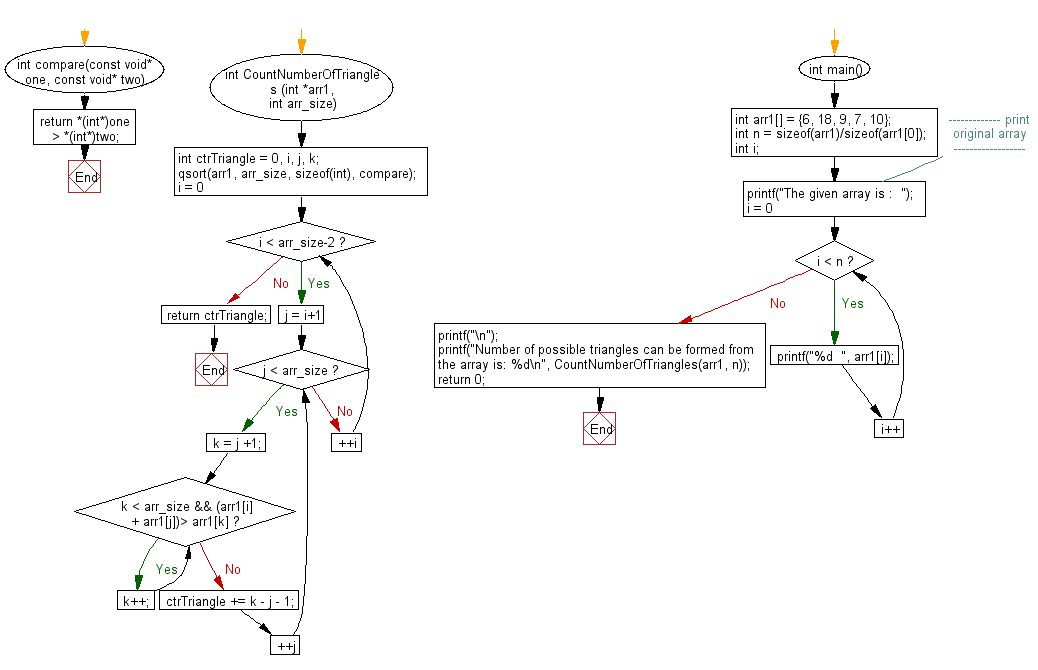﻿ C exercises: Count the number of triangles can be fromed from a given array - w3resource# C Exercises: Count the number of triangles can be fromed from a given array

## C Array: Exercise-52 with Solution

Write a program in C to count the number of triangles can be fromed from a given array.

Sample Solution:

C Code:

``````#include <stdio.h>
#include <stdlib.h>

int compare(const void* one, const void* two)
{
return *(int*)one > *(int*)two;
}
int CountNumberOfTriangles (int *arr1, int arr_size)
{
int ctrTriangle = 0, i, j, k;
qsort(arr1, arr_size, sizeof(int), compare);

for(i = 0; i < arr_size-2; ++i)
{

for (j = i+1; j < arr_size; ++j)
{
k = j +1;
while (k < arr_size && (arr1[i] + arr1[j])> arr1[k])
{
k++;
}
ctrTriangle += k - j - 1;
}
}
return ctrTriangle;
}
int main()
{
int arr1[] =  {6, 18, 9, 7, 10};
int n = sizeof(arr1)/sizeof(arr1);
int i;
//------------- print original array ------------------
printf("The given array is :  ");
for(i = 0; i < n; i++)
{
printf("%d  ", arr1[i]);
}
printf("\n");
//------------------------------------------------------
printf("Number of possible triangles can be formed from the array is: %d\n",
CountNumberOfTriangles(arr1, n));
return 0;
}
```
```

Sample Output:

```The given array is :  6  18  9  7  10
Number of possible triangles can be formed from the array is: 5
```

Flowchart:C Programming Code Editor:

Improve this sample solution and post your code through Disqus.

What is the difficulty level of this exercise?

﻿